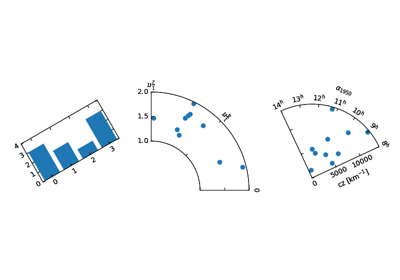/Matplotlib 3.0

# mpl_toolkits.axisartist.floating_axes.GridHelperCurveLinear

`class mpl_toolkits.axisartist.floating_axes.GridHelperCurveLinear(aux_trans, extremes, grid_locator1=None, grid_locator2=None, tick_formatter1=None, tick_formatter2=None)` [source]

aux_trans : a transform from the source (curved) coordinate to target (rectilinear) coordinate. An instance of MPL's Transform (inverse transform should be defined) or a tuple of two callable objects which defines the transform and its inverse. The callables need take two arguments of array of source coordinates and should return two target coordinates: e.g., x2, y2 = trans(x1, y1)

`get_boundary()` [source]

return Nx2 array of x,y coordinate of the boundary

`get_data_boundary(side)` [source]

return v= 0 , nth=1

`get_gridlines(which='major', axis='both')` [source]

Return list of grid lines as a list of paths (list of points).

which : "major" or "minor" axis : "both", "x" or "y"

`new_fixed_axis(loc, nth_coord=None, axis_direction=None, offset=None, axes=None)` [source]

## Examples using `mpl_toolkits.axisartist.floating_axes.GridHelperCurveLinear`Demo Floating Axes